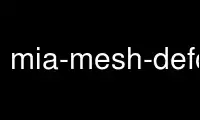# mia-mesh-deformable-model - 云端在线### 程序：

#### 名称

mia-mesh-deformable-model - 使用可变形模型拟合网格。

#### 概要

mia-mesh-deformable-model -i -o -r [选项]

#### 商品描述

mia-mesh-deformable-model 该程序运行一个可变形模型来使网格适应等

#### 配置

-i --in-file=（输入，必填）； io

-o --out-file=（输出，需要）； io

-r --ref-file=（输入，必填）； io

-V --verbose=警告

info - 低级消息

message - 普通消息

- 版权

-h --帮助

-？ - 用法

- 版

--平滑权重=0.04； 浮动在 [0, inf)

--梯度权重=0.04

--强度-权重=0.02； 浮动在 [0, inf)

--intensity-scaling=1; 浮在 (0, inf)

-s --iso=64

verices 应该适应。

--图像平滑=高斯：w=2

--线程=-1

number 应该小于或等于逻辑处理器内核的数量

-m --maxiter=200; 输入 (0, inf)

-e --epsilon=0.001; 浮在 (0, inf)

--重定向

#### 插件：1d/空间内核

（无参数）

w = 1; 输入 [0, inf)

（无参数）

（无参数）

（无参数）

#### 插件：一维/样条内核

d = 3; [0, 5] 中的整数

d = 3; [3, 3] 中的整数

（无参数）

（无参数）

DIV 图像组合器“div”

（无参数）

MUL 图像组合器“mul”

（无参数）

（无参数）

#### 插件：3d图像/过滤器

false，第一个操作符是通过过滤器管道的图像，以及

op =（必需，工厂）

a = 1; 漂浮

b = 0; 漂浮

- 有符号的 32 位

- 有符号的 16 位
UINT - 无符号 32 位

- 二进制数据

（无参数）

b = [[1,1,1]]; 3d边界

bx = 1; 输入 [1, inf)
x 方向的块大小。

by = 1; 输入 [1, inf)
y 方向的块大小。

bz = 1; 输入 [1, inf)
z 方向的块大小。

w = 1; int in [0, inf)

（无参数）

（无参数）

c = 3; int in [2, inf)

n = 6n; 工厂

lvdownscale

b = [[1,1,1]]; 3d边界

w = 1; int in [1, inf)

w = 1; int in [1, inf)

w = 1; int in [1, inf)

3D图像mean-sigma归一化滤波器，支持的参数有：

w = 1; int in [1, inf)

p-zxy - 置换 x->y->z->x
r-x180 - 绕 x 轴顺时针旋转 180 度
XYZ - 保持方向
yzx - 置换 x->z->y->x
r-z180 - 绕z轴顺时针旋转180度
r-y270 - 绕y轴顺时针旋转270度
f-xz - 翻转 xz
f-yz - 翻转 yz
r-x90 - 绕 x 轴顺时针旋转 90 度
r-y90 - 绕y轴顺时针旋转90度
r-x270 - 绕 x 轴顺时针旋转 270 度
r-z270 - 绕z轴顺时针旋转270度
r-z90 - 绕z轴顺时针旋转90度
f-xy - 翻转 xy
r-y180 - 绕y轴顺时针旋转180度

w = 1; int in [1, inf)

s = [[0,0,0]]; 3d边界

sx = 0; 输入 [0, inf)
x 方向的目标大小（0：使用输入图像大小）。

sy = 0; 输入 [0, inf)
y 方向的目标大小（0：使用输入图像大小）。

sz = 0; 输入 [0, inf)
y 方向的目标大小（0：使用输入图像大小）。

（无参数）

sepconv 3D图像强度分离卷积滤波器，支持的参数有：

kx = [高斯：w=1]； 工厂

ky = [高斯：w=1]； 工厂

kz = [高斯：w=1]； 工厂
z 方向的滤波器内核。 有关支持的插件，请参阅

SWS 种子水源。 该算法提取的区域正好与初始区域一样多

n = [球体：r=1]； 工厂

（无参数）

w = 1; int in [1, inf)

ws 基本水源分割，支持的参数有：

n = [球体：r=1]； 工厂

#### 插件：二维图像/IO

HDF5 HDF5 3D图像IO

MHD MetaIO 3D 图像 IO 使用 VTK 实现（实验性）。

vti 3D 图像 VTK-XML 输入和输出（实验性）。

#### 插件：3d图像/形状

18n 18n 邻域 3D 形状创建器

（无参数）

26n 26n 邻域 3D 形状创建器

（无参数）

6n 6n 邻域 3D 形状创建器

（无参数）

r.，支持的参数有：

r = 2; 浮在 (0, inf)

#### 插件：3d变换/io

BBS 3D 转换的二进制（不可移植）序列化 IO

XML 3D 转换的 XML 序列化 IO

STL STL网格io插件

#### 例

mia-mesh-deformable-model -i input.vmesh -o 变形.vmesh --iso 128 --maxiter 200